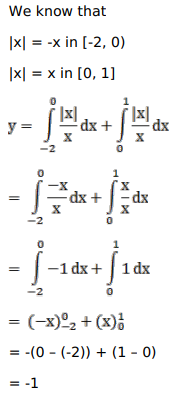# Solve this following

Question:

Mark $(\sqrt{ })$ against the correct answer in the following:

$\int_{-2}^{1} \frac{|\mathrm{x}|}{\mathrm{x}} \mathrm{dx}=?$

A. 3

B. $2.5$

C. $1.5$

D. none of these

Solution: